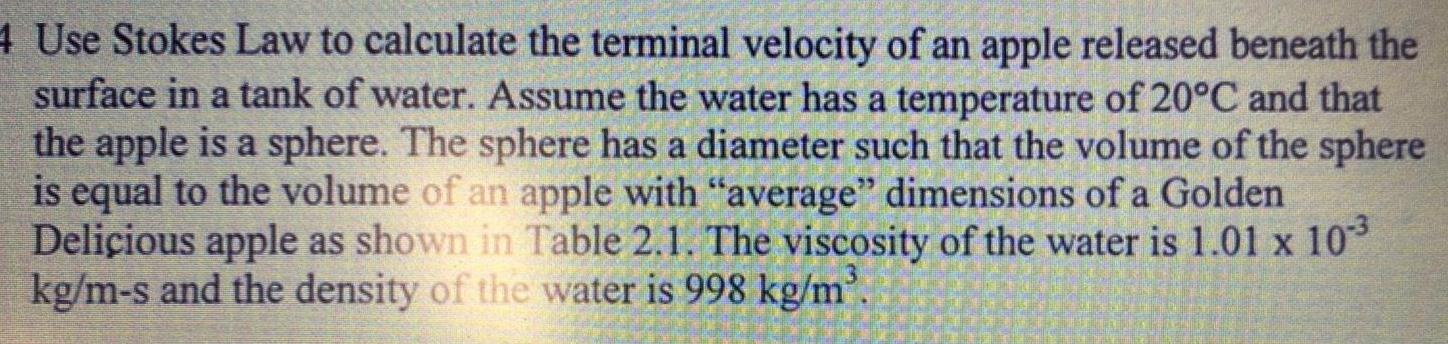Question:

# 4 Use Stokes Law to calculate the terminal velocity of an

Last updated: 11/20/20234 Use Stokes Law to calculate the terminal velocity of an apple released beneath the surface in a tank of water Assume the water has a temperature of 20 C and that the apple is a sphere The sphere has a diameter such that the volume of the sphere is equal to the volume of an apple with average dimensions of a Golden Delicious apple as shown in Table 2 1 The viscosity of the water is 1 01 x 10 kg m s and the density of the water is 998 kg m